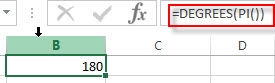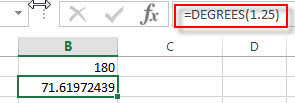# Excel DEGREES Function

This post will guide you how to use Excel DEGREES function with syntax and examples in Microsoft excel.

### Description

The Excel DEGREES function converts radians into degrees. And it will return a numeric value. For examples, if you pass the PI() function into the DEGREES function as its argument, and it will return 180.

The DEGREES function is a build-in function in Microsoft Excel and it is categorized as a Math and Trigonometry Function.

The DEGREES function in Excel 2016, Excel 2013, Excel 2010, Excel 2007, Excel 2003, Excel XP, Excel 2000, Excel 2011 for Mac.

### Syntax

The syntax of the DEGREES function is as below:

`=DEGREES (angle)`

Where the DEGREES function arguments is:

• angle -This is a required argument. .The angle in radians that you want to convert to degrees.

### Excel DEGREES Function Examples

The below examples will show you how to use Excel DEGREES Function to convert radians into degrees.

1# get the degrees of pi radians, enter the following formula in Cell B1.

`=DEGREES (PI())`2# get the degrees of 1.25 radians, enter the following formula in Cell B2.

`=DEGREES (1.25)`### Related Functions

• Excel PI function
The Excel PI function returns the value of mathematical constant PI. The returned value is 3.14159265358979 and ti will accurate to 15 digits.The syntax of the PI function is as below:=PI()…